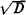# Pell's Equation

Also found in: Wikipedia.

## Pell’s Equation

an equation of the form x2 - Dy2 = 1, where D is a positive integer that is not a perfect square and the equation is to be solved in integers. The equation has an infinite number of solutions. The solution x0 = 1, y0 = 0 is obvious. The next—in terms of magnitude—solution (x1, y1) of Pell’s equation can be found by expandinginto a continued fraction. If we know the solution (x1, y1), the entire set of solutions (xn yn) can be obtained by using the formulaPell’s equation is closely related to the theory of algebraic numbers. It is named after the 17th-century British mathematician J. Pell, to whom L. Euler incorrectly attributed a method of solving this equation.

### REFERENCES

Venkov, B. A. Elementarnaia teoriia chisel. Moscow-Leningrad, 1937. Chapter 2.
Dickson, L. E. History of the Theory of Numbers, vol. 2. New York, 1966.
Mentioned in ?
References in periodicals archive ?
In this paper, we show that the problem of finding the Smarandache friendly pairs can be reduced to solving a particular type of Pell's equation, which can then be used to find the sequence of all Smarandache friendly pairs.
The general solution to Pell's equation was first obtained by Lagrange who presented a number of papers on this topic to the Berlin Academy between 1768 and 1770.
Four reference appendices are included, on solving Pell's equation, geometrical inversion, basic facts from graph theory, and linear difference equations with constant coefficients.
After a fine introduction to basic notions, he covers unique factorization, the Gaussian integers, and Pell's equation, and moves on to algebraic number theory.
In this paper is considered the Pell's equation [x.
Keywords Pell's equation, solutions of Pell's equation.
There are many papers in which different types of Pell's equation are considered (see , , , , , ).
1]) be the fundamental solution of the Pell's equation [x.
Then the integer solutions of the Pell's equation [x.
1]) is the fundamental solution of the Pell's equation [x.
1]) is the fundamental solutions of the negative Pell's equation [x.
Ramasamy, The characteristic number of two simultaneous Pell's equations and its application, Simon Stevin 59 (1985),203214.

Site: Follow: Share:
Open / Close